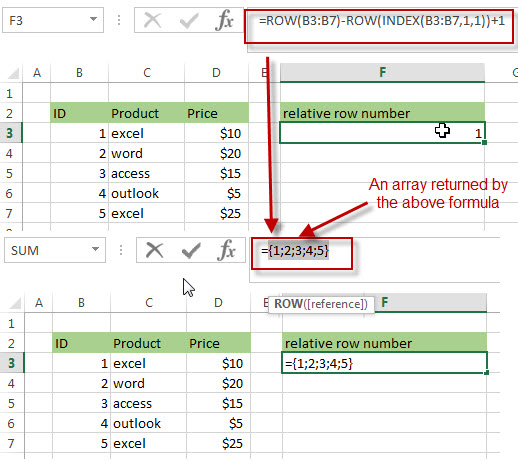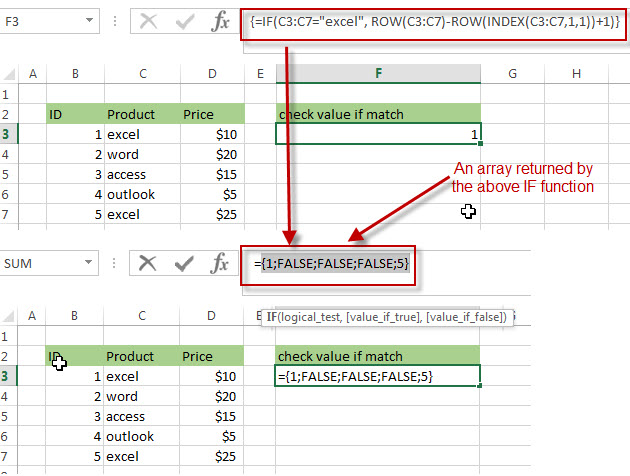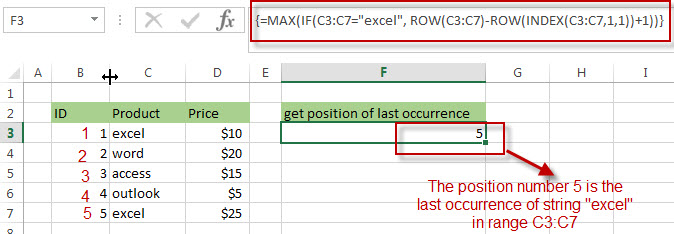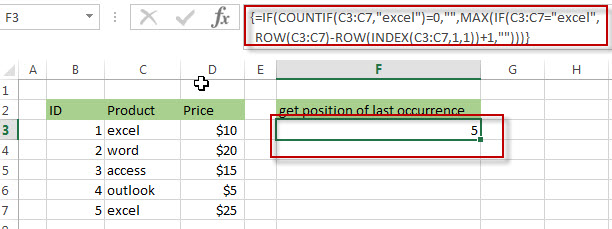# How to get the position of Last Occurrence of a value in a column

This post explains that how to get the position of the last occurrence of a specific value in a column in excel. Is there a formula or function that finds the last match of a specific value in a column in excel 2013 or 2016? The below will guide you how to find the position of the last occurrence of a given value in a column.

## Get the position (index) of Last Occurrence of a value

If you want to find the position number of the last occurrence of a specific value in a column (a single range), you can use an array formula with a combination of the MAX function, IF function, ROW function and INDEX Function.

Suppose that you want to find the position of the last occurrence of a text string as “excel” in a range C3:C7, you can try to use the following array formulas:

`=MAX(IF(C3:C7="excel", ROW(C3:C7)-ROW(INDEX(C3:C7,1,1))+1))`

Note: when you enter the above array formula, you must hold the Control and Shift keys down, then press Enter. Then the formula bar should add curly braces surrounding the array formula.

Let’s see how the above formula works:

=ROW(C3:C7)-ROW(INDEX(C3:C7,1,1))+1

I have talked the above formula in the previous post, it returns the relative position of all rows in the range B3:B7. The result returned by the above formula is an array.=IF(C3:C7=”excel”, ROW(C3:C7)-ROW(INDEX(C3:C7,1,1))+1)

The IF function will check if each value in range B3:B7 is equal to “excel”, if TRUE, returns its relative position, otherwise, returns FALSE. As this formula is an array formula, so it must be returned an array result.=MAX(IF(C3:C7=”excel”, ROW(C3:C7)-ROW(INDEX(C3:C7,1,1))+1))

The MAX function will check the array result returned by the IF function and returns the highest value in the array. So it should be the position of the last occurrence of text string “excel” in range B3:B7. It is 5.You can also use the below two array formula to achieve the same results:

The first array formula:

`=IF(COUNTIF(C3:C7,"excel")=0,"",MAX(IF(C3:C7="excel",ROW(C3:C7)-ROW(C3)+1,"")))`

The COUNTIF function will count the number of cells in the range C3:C7 that equal to the string “excel”.  The First IF function will return an empty string if the result returned by the COUNTIF function is FALSE. Or returns the position number of the last occurrence of string “excel” in the range C3:C7.The second array formula:

`=MAX(IF(C3:C7="excel", ROW(C3:C7)-MIN(ROW(C3:C7))+1))`### Related Formulas

• Get the Position of the nth Occurrence of a Character in a Cell
If you want to get the position of the nth occurrence of a character using a excel formula, you can use the FIND function in combination with the SUBSTITUTE function.
•  Get the position of Last Occurrence of a character or string in a cell
If you want to get the position of the last occurrence of a character in a cell, then you can use a combination of the LOOKUP function, the MID function, the ROW function, the INDIRECT function and the LEN function to create an excel formula.…
• Split Text String to an Array
If you want to convert a text string into an array that split each character in text as an element, you can use an excel formula to achieve this result. the below will guide you how to use a combination of the MID function, the ROW function, the INDIRECT function and the LEN function to split a string…
• Find the Relative Position in a Range or Table
If you want to know the relative row position for all rows in an Excel Range (B3:D6), you can use a excel Array formula as follows:=ROW(B3:D6)- ROW(B3) + 1. You can also use another excel array formula to get the same result as follows:=ROW(B3:D6)-ROW(INDEX(B3:D6,1,1))+1…
• Get the First Row Number in a Range
If the ROW function use a Range as its argument, it only returns the first row number.You can also use the ROW function within the MIN function to get the first row number in a range. You can also use the INDEX function to get the reference of the first row in a range, then combined to the ROW function to get the first row number of a range.…
•  Get the Last Row Number in a Range
If you want to get the last row number in a range, you need to know the first row number and the total rows number of a range, then perform the addition operation, then subtract 1, the last result is the last row number for that range.…

### Related Functions

• Excel INDEX function
The Excel INDEX function returns a value from a table based on the index (row number and column number)The INDEX function is a build-in function in Microsoft Excel and it is categorized as a Lookup and Reference Function.The syntax of the INDEX function is as below:= INDEX (array, row_num,[column_num])…
• Excel ROW function
The Excel ROW function returns the row number of a cell reference.The ROW function is a build-in function in Microsoft Excel and it is categorized as a Lookup and Reference Function.The syntax of the ROW function is as below:= ROW ([reference])….
• Excel MIN function
The Excel MIN function returns the smallest numeric value from the numbers that you provided. Or returns the smallest value in the array.The MIN function is a build-in function in Microsoft Excel and it is categorized as a Statistical Function.The syntax of the MIN function is as below:= MIN(num1,[num2,…numn])….
• Excel IF function
The Excel IF function perform a logical test to return one value if the condition is TRUE and return another value if the condition is FALSE. The IF function is a build-in function in Microsoft Excel and it is categorized as a Logical Function.The syntax of the IF function is as below:= IF (condition, [true_value], [false_value])….
• Excel COUNTIF function
The Excel COUNTIF function will count the number of cells in a range that meet a given criteria.This function can be used to count the different kinds of cells with number, date, text values, blank, non-blanks, or containing specific characters.etc.The syntax of the COUNTIF function is as below:= COUNTIF (range, criteria) …
• Excel MAX function
The Excel MAX function returns the largest numeric value from the numbers that you provided. Or returns the largest value in the array.= MAX(num1,[num2,…numn])…